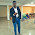### PACKED AND UNPACKED BINARY CODED DECIMAL (BCD) NUMBERS

WHAT ARE PACKED AND UNPACKED BINARY CODED DECIMAL (BCD) NUMBERS

In the case of unpacked BCD numbers, each four-bit BCD group corresponding to a decimal digit is stored in a separate register inside the machine. In such a case, if the registers are eight bits or wider, the register space is wasted.

In the case of packed BCD numbers, two BCD digits are stored in a single eight-bit register. The process of combining two BCD digits so that they are stored in one eight-bit register involves shifting the number in the upper register to the left 4 times and then adding the numbers in the upper and lower registers.

The process is illustrated by showing the storage of decimal digits ‘5’ and ‘7’:

• Decimal digit 5 is initially stored in the eight-bit register as: 0000 0101.

• Decimal digit 7 is initially stored in the eight-bit register as: 0000 0111.

• After shifting to the left 4 times, the digit 5 register reads: 0101 0000.

• The addition of the contents of the digit 5 and digit 7 registers now reads: 0101 0111.

Example
How many bits would be required to encode decimal numbers 0 to 9999 in straight binary and BCD codes? What would be the BCD equivalent of decimal 27 in 16-bit representation?

Solution

• Total number of decimals to be represented=10 000=104 =213 29.

• Therefore, the number of bits required for straight binary encoding=14.

• The number of bits required for BCD encoding=16.

• The BCD equivalent of 27 in 16-bit representation=0000000000100111.

1.thank you very much

2.3.4.5.